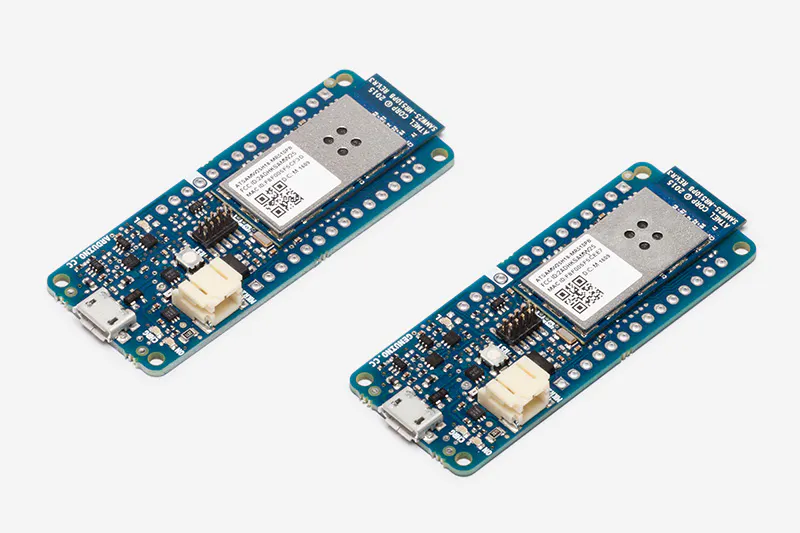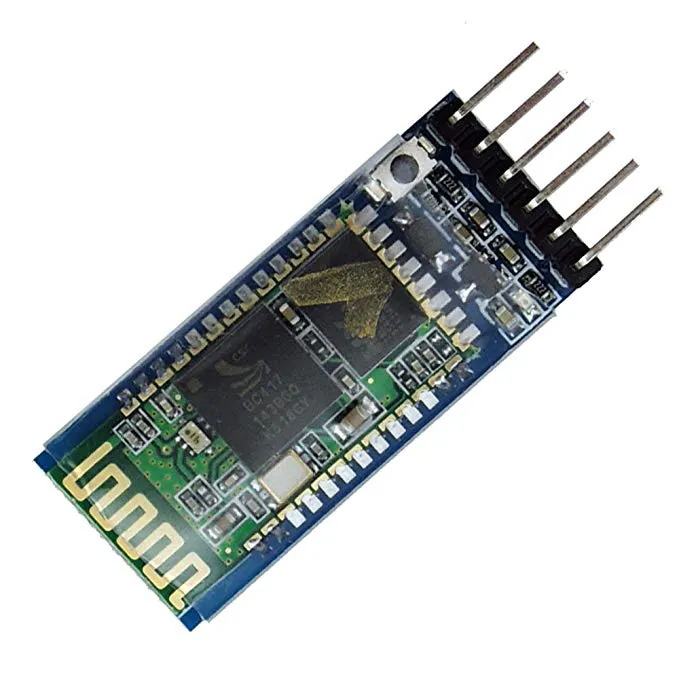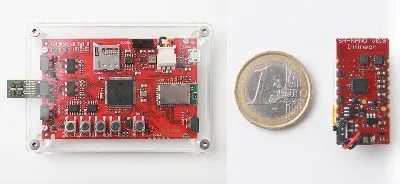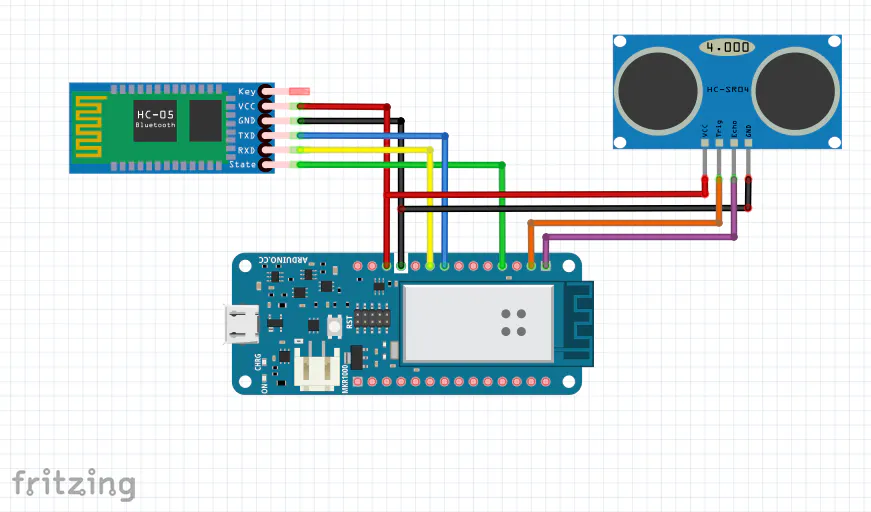Autonomous Delivery System

An Autonomous Delivery System using the DonkeyCar which allows you to send or receive physical items, in a number of different scenarios.

Things used in this project

Hardware components

 DonkeyCar Kit
×1Arduino MKR1000
×1Ultrasonic Sensor - HC-SR04 (Generic)
×1HC-05 Bluetooth Module
×1Infineon Sensor Hub Nano
×1

Software apps and online servicesTensorFlowArduino IDE

Hand tools and fabrication machinesSoldering iron (generic)Hot glue gun (generic)

Schematics

MKR1000 with ultrasonic sensor and bluetooth module

Fritzing diagram of the Arduino MKR1000 with the ultrasonic sensor and bluetooth module. Just wire everything according to this.Code

MKR1000 Ultrasonic Sensor and Beacon

Arduino
This is the final code you will need to upload to the MKR1000 before you connect it to the Raspberry Pi. Make sure to replace the minimumValue and maximumValue to what you found!
//Replace these with minimum and values you found
int minimumValue = 6;
int maximumValue = 12;

int trigPin = 7;      //Trigger pin
int echoPin = 6;      //Echo pin
int btStatePin = 9;   //Bluetooth state pin
int isLetterDetected;
long duration, cm;
byte message;

// Define the number of samples to keep track of. The higher the number, the
// more the readings will be smoothed, but the slower the output will respond to
// the input. Using a constant rather than a normal variable lets us use this
// value to determine the size of the readings array.

int total = 0;                  // the running total
int average = 0;                // the average

//BTconnected is false when not connected and true when connected
boolean BTconnected = false;

void setup() {
// initialize serial communication with computer:
Serial.begin(9600);
// initialize all the readings to 0:
}
//Define inputs and outputs
pinMode(trigPin, OUTPUT);
pinMode(echoPin, INPUT);
pinMode(btStatePin, INPUT);
}

void loop() {
// The sensor is triggered by a HIGH pulse of 10 or more microseconds.
// Give a short LOW pulse beforehand to ensure a clean HIGH pulse:
digitalWrite(trigPin, LOW);
delayMicroseconds(5);
digitalWrite(trigPin, HIGH);
delayMicroseconds(10);
digitalWrite(trigPin, LOW);

// Read the signal from the sensor: a HIGH pulse whose
// duration is the time (in microseconds) from the sending
// of the ping to the reception of its echo off of an object.
pinMode(echoPin, INPUT);
duration = pulseIn(echoPin, HIGH);

// Convert the time into a distance
cm = (duration / 2) / 29.1;   // Divide by 29.1 or multiply by 0.0343

// advance to the next position in the array:

// if we're at the end of the array...
// ...wrap around to the beginning:
}

// calculate the average:
// send it to the computer as ASCII digits

if (average >= minimumValue && average <= maximumValue) {
isLetterDetected = 1;
}
else {
isLetterDetected = 0;
}

BTconnected = true;
}
else {
BTconnected = false;
}

if (message == '1') {
Serial.print(isLetterDetected);
Serial.print(":");
Serial.println(BTconnected);
}

delay(30);
}

Ultrasonic sensor with MKR1000

Arduino
This will allow you to test the ultrasonic sensor with the MKR1000. It finds the distance, and prints an average value of the distance in centimeters
int trigPin = 7;    // Trigger
int echoPin = 6;    // Echo
long duration, cm;

int total = 0;                  // the running total
int averagecm = 0;

void setup() {
//Serial Port begin
Serial.begin(9600);
//Define inputs and outputs
pinMode(trigPin, OUTPUT);
pinMode(echoPin, INPUT);
// initialize all the readings to 0:
}
}

void loop() {
// The sensor is triggered by a HIGH pulse of 10 or more microseconds.
// Give a short LOW pulse beforehand to ensure a clean HIGH pulse:
digitalWrite(trigPin, LOW);
delayMicroseconds(5);
digitalWrite(trigPin, HIGH);
delayMicroseconds(10);
digitalWrite(trigPin, LOW);

// Read the signal from the sensor: a HIGH pulse whose
// duration is the time (in microseconds) from the sending
// of the ping to the reception of its echo off of an object.
pinMode(echoPin, INPUT);
duration = pulseIn(echoPin, HIGH);

// Convert the time into a distance
cm = (duration / 2) / 29.1;   // Divide by 29.1 or multiply by 0.0343

// advance to the next position in the array:

// if we're at the end of the array...
// ...wrap around to the beginning:
}

// calculate the average:
// send it to the computer as ASCII digits

Serial.println(averagecm);

delay(100);
}

Modified manage.py

Python
This is the modified manage.py file. It needs to be replaced with the manage.py file in the mycar directory, and needs to have the same name (manage.py)
#!/usr/bin/env python3
"""
Scripts to drive a donkey 2 car and train a model for it.

Usage:
manage.py (drive) [--model=<model>] [--js] [--chaos]
manage.py (train) [--tub=<tub1,tub2,..tubn>]  (--model=<model>) [--base_model=<base_model>] [--no_cache]

Options:
-h --help        Show this screen.
--tub TUBPATHS   List of paths to tubs. Comma separated. Use quotes to use wildcards. ie "~/tubs/*"
--js             Use physical joystick.
--chaos          Add periodic random steering when manually driving
"""
import os
from docopt import docopt

import donkeycar as dk

#import parts
from donkeycar.parts.camera import PiCamera
from donkeycar.parts.transform import Lambda
from donkeycar.parts.keras import KerasCategorical
from donkeycar.parts.actuator import PCA9685, PWMSteering, PWMThrottle
from donkeycar.parts.datastore import TubGroup, TubWriter
from donkeycar.parts.controller import LocalWebController, JoystickController
from donkeycar.parts.clock import Timestamp
from MKR1000 import MKR1000Serial

def drive(cfg, model_path=None, use_joystick=False, use_chaos=False):
"""
Construct a working robotic vehicle from many parts.
Each part runs as a job in the Vehicle loop, calling either
All parts are updated one after another at the framerate given in
cfg.DRIVE_LOOP_HZ assuming each part finishes processing in a timely manner.
Parts may have named outputs and inputs. The framework handles passing named outputs
to parts requesting the same named input.
"""

V = dk.vehicle.Vehicle()

mkr1000 = MKR1000Serial()

clock = Timestamp()

cam = PiCamera(resolution=cfg.CAMERA_RESOLUTION)

if use_joystick or cfg.USE_JOYSTICK_AS_DEFAULT:
ctr = JoystickController(max_throttle=cfg.JOYSTICK_MAX_THROTTLE,
steering_scale=cfg.JOYSTICK_STEERING_SCALE,
auto_record_on_throttle=cfg.AUTO_RECORD_ON_THROTTLE)
else:
# This web controller will create a web server that is capable
# of managing steering, throttle, and modes, and more.
ctr = LocalWebController(use_chaos=use_chaos)

inputs=['cam/image_array'],
outputs=['user/angle', 'user/throttle', 'user/mode', 'recording'],

# See if we should even run the pilot module.
# This is only needed because the part run_condition only accepts boolean
def pilot_condition(mode):
if mode == 'user':
return False
else:
return True

pilot_condition_part = Lambda(pilot_condition)
outputs=['run_pilot'])

# Run the pilot if the mode is not user.
kl = KerasCategorical()
if model_path:

outputs=['pilot/angle', 'pilot/throttle'],
run_condition='run_pilot')

# Choose what inputs should change the car.
def drive_mode(mode,
user_angle, user_throttle,
pilot_angle, pilot_throttle):
if mode == 'user':
return user_angle, user_throttle

elif mode == 'local_angle':
return pilot_angle, user_throttle

else:
return pilot_angle, pilot_throttle

drive_mode_part = Lambda(drive_mode)
inputs=['user/mode', 'user/angle', 'user/throttle',
'pilot/angle', 'pilot/throttle'],
outputs=['angle', 'throttle'])

steering_controller = PCA9685(cfg.STEERING_CHANNEL)
steering = PWMSteering(controller=steering_controller,
left_pulse=cfg.STEERING_LEFT_PWM,
right_pulse=cfg.STEERING_RIGHT_PWM)

throttle_controller = PCA9685(cfg.THROTTLE_CHANNEL)
throttle = PWMThrottle(controller=throttle_controller,
max_pulse=cfg.THROTTLE_FORWARD_PWM,
zero_pulse=cfg.THROTTLE_STOPPED_PWM,
min_pulse=cfg.THROTTLE_REVERSE_PWM)

# add tub to save data
inputs = ['cam/image_array', 'user/angle', 'user/throttle', 'user/mode', 'timestamp']
types = ['image_array', 'float', 'float',  'str', 'str']

#multiple tubs
#th = TubHandler(path=cfg.DATA_PATH)
#tub = th.new_tub_writer(inputs=inputs, types=types)

# single tub
tub = TubWriter(path=cfg.TUB_PATH, inputs=inputs, types=types)

V.start(rate_hz=cfg.DRIVE_LOOP_HZ,
max_loop_count=cfg.MAX_LOOPS)

def train(cfg, tub_names, new_model_path, base_model_path=None ):
"""
use the specified data in tub_names to train an artifical neural network
saves the output trained model as model_name
"""
X_keys = ['cam/image_array']
y_keys = ['user/angle', 'user/throttle']
def train_record_transform(record):
""" convert categorical steering to linear and apply image augmentations """
record['user/angle'] = dk.util.data.linear_bin(record['user/angle'])
# TODO add augmentation that doesn't use opencv
return record

def val_record_transform(record):
""" convert categorical steering to linear """
record['user/angle'] = dk.util.data.linear_bin(record['user/angle'])
return record

new_model_path = os.path.expanduser(new_model_path)

kl = KerasCategorical()
if base_model_path is not None:
base_model_path = os.path.expanduser(base_model_path)

print('tub_names', tub_names)
if not tub_names:
tub_names = os.path.join(cfg.DATA_PATH, '*')
tubgroup = TubGroup(tub_names)
train_gen, val_gen = tubgroup.get_train_val_gen(X_keys, y_keys,
train_record_transform=train_record_transform,
val_record_transform=val_record_transform,
batch_size=cfg.BATCH_SIZE,
train_frac=cfg.TRAIN_TEST_SPLIT)

total_records = len(tubgroup.df)
total_train = int(total_records * cfg.TRAIN_TEST_SPLIT)
total_val = total_records - total_train
print('train: %d, validation: %d' % (total_train, total_val))
steps_per_epoch = total_train // cfg.BATCH_SIZE
print('steps_per_epoch', steps_per_epoch)

kl.train(train_gen,
val_gen,
saved_model_path=new_model_path,
steps=steps_per_epoch,
train_split=cfg.TRAIN_TEST_SPLIT)

if __name__ == '__main__':
args = docopt(__doc__)

if args['drive']:
drive(cfg, model_path = args['--model'], use_joystick=args['--js'], use_chaos=args['--chaos'])

elif args['train']:
tub = args['--tub']
new_model_path = args['--model']
base_model_path = args['--base_model']
cache = not args['--no_cache']
train(cfg, tub, new_model_path, base_model_path)

MKR1000.py

Python
This is the python file for the custom MKR1000 part for the DonkeyCar. You need to edit your MKR1000's serial port in the Raspberry Pi, and place it in the mycar directory.
import serial
import time

class MKR1000Serial:
def update(self):
print('Starting MKR1000 serial connection...')
ser = serial.Serial('/dev/ttyACM0', 9600)
ser.write(b"1")
time.sleep(0.0625)
if(ser.in_waiting >0):